# PSAT Math : Factoring and Simplifying Square Roots

## Example Questions

← Previous 1

### Example Question #1 : Basic Squaring / Square Roots

Solve for: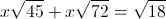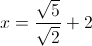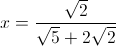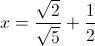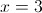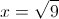Explanation:Notice how all of the quantities in square roots are divisible by 9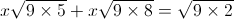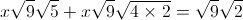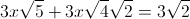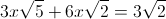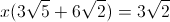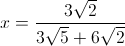Simplifying, this becomes### Example Question #1 : How To Find The Common Factors Of Squares

If m and n are postive integers and 4m = 2n, what is the value of m/n?

2

8

4

1/2

16

1/2

Explanation:
1. 2= 4. Also, following the rules of exponents, 4= 1.
2. One can therefore say that m = 1 and n = 2.
3. The question asks to solve for m/n. Since m = 1 and n = 2, m/n = 1/2.

### Example Question #1 : Factoring And Simplifying Square Roots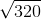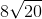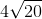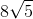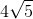Explanation: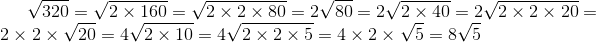### Example Question #2 : Factoring And Simplifying Square Roots

Simplify. Assume all variables are positive real numbers.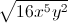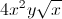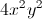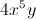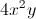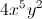Explanation:The index coefficent in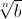is represented by. When no index is present, assume it is equal to 2.under the radical is known as the radican, the number you are taking a root of.

First look for a perfect square,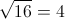Then to your Variables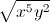Take your exponents on both variables and determine the number of times our index will evenly go into both.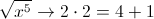So you would take out a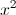and would be left with a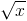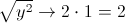*Dividing the radican exponent by the index - gives you the number of variables that should be pulled out.

The final answer would be.

### Example Question #1 : How To Simplify Square Roots

Simplify. Assume all integers are positive real numbers.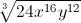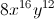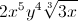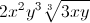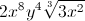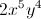Explanation:Index ofmeans the cube root of Radican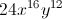Find a perfect cube in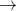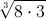Simplify the perfect cube, giving you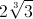.

Take your exponents on both variables and determine the number of times our index will evenly go into both.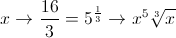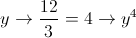### Example Question #2 : Factoring And Simplifying Square Roots

Simplify square roots. Assume all integers are positive real numbers.

Simplify as much as possible. List all possible answers.

1a.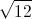1b.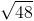1c.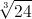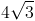and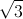and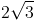andandandandand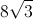andandExplanation:

When simplifying radicans (integers under the radical symbol), we first want to look for a perfect square. For example,is not a perfect square. You look to find factors ofto see if there is a perfect square factor in, which there is.

1a.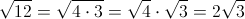Do the same thing for.

1b.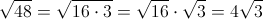1c.Follow the same procedure except now you are looking for perfect cubes.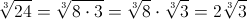### Example Question #1 : How To Simplify Square Roots

Simplify

÷ √3

2

not possible

3

3√3

none of these

3√3

Explanation:

in order to simplify a square root on the bottom, multiply top and bottom by the root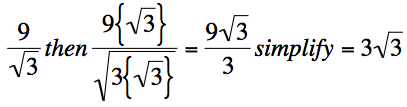### Example Question #1 : How To Simplify Square Roots

Simplify:

√112

4√7

20

10√12

4√10

12

4√7

Explanation:

√112 = {√2 * √56} = {√2 * √2 * √28} = {2√28} = {2√4 * √7} = 4√7

### Example Question #1 : How To Simplify Square Roots

Simplify:

√192

None of these
8√2
4√2
8√3
4√3
Explanation:

√192 = √2 X √96

√96 = √2 X √48

√48 = √4 X√12

√12 = √4 X √3

√192 = √(2X2X4X4) X √3

= √4X√4X√4  X √3

= 8√3

### Example Question #2 : How To Simplify Square Roots

What is the simplest way to express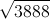?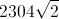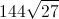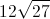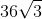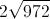Explanation:

First we will list the factors of 3888: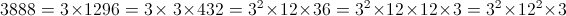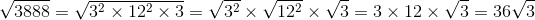← Previous 1

### All PSAT Math Resources